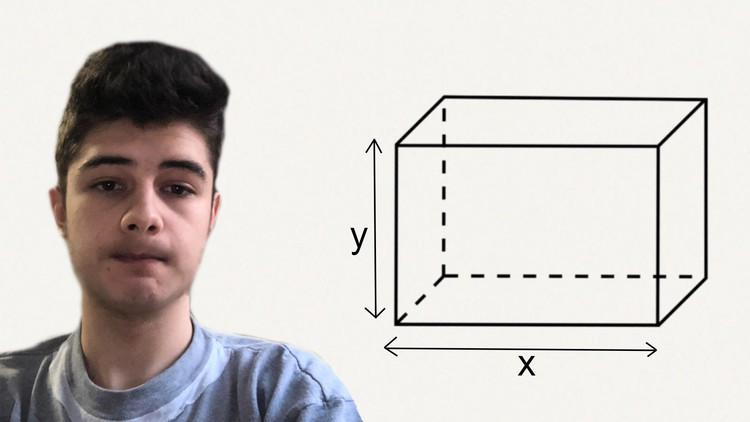# Learn Geometry: geometry for beginnersWhat you will learn

### Solve problems using the criteria you’ll learn in this course

Description

Learn Geometry: geometry for beginners

This course will teach you basic to intermediate geometry, in less then 3 hours. The course includes lots of quizzes so that you can test your knowledge + one practice test with geometry problems and questions.

My course will explain as concise as possible the most important concepts of basic and intermediate geometry, so that anyone can grasp the information. I made the course smaller, so that if you will ever need to remember some criteria you have forgotten, you can come back and take this geometry course which will be the best refresh you’ll ever need.

What will students learn?

1. Types of shapes: Quadrilaterals, triangles, and circles
2. Parallel, Secant and Perpendicular lines
3. Types of angles: Acute, Obtuse, Right
4. Alternate-exterior / Alternate-interior angles
5. Triangle congruence by the criteria: SSS, ASA, SAS
6. Triangle similarity by the criteria: AA, SSS, SAS
7. Pythagorean theorem
8. Criteria for: Square, Rectangle, Right triangle, Equilateral triangle, Isosceles triangle, Circle, Parallelogram, Rhombus, Trapezoid.
9. Solve problems using the criteria you’ll learn in this course

• Anyone who is willing to learn geometry

I tried to make this course as concise as possible, so it’s not too long, but still it gives you enough time to comprehend and process the information it gives you.

I look forward to seeing you in my lectures, so that we can explore more deep the secrets of geometry!

Marius Manola

English
language

Content

### Introduction

Lines, segments & rays
Lines, segments & rays
Alternate angles
Alternate angles
Types of Triangles
Types of Triangles

### Intermediate Geometry – for triangles

Median, Bisector and Altitude
Median, Bisector and Altitude
Exercises
The Isosceles triangle
The Isosceles triangle
The Equilateral triangle
The Equilateral triangle
The Right triangle
The Right triangle
Congruent Triangle
Thales’s theorem
Similarity of Triangles
Problems

### The basics for the Circle

The Circle
The circle
Circle problem

The Square
The Rectangle
The rectangle
Rectangle problem
The Parallelogram
The Parallelogram
Parallelogram problem
The Diamond / Rhombus
The Rhombus
Rhombus problem
The Trapezoid
The Trapezoid
Trapezoid problem
Bonus Lecture